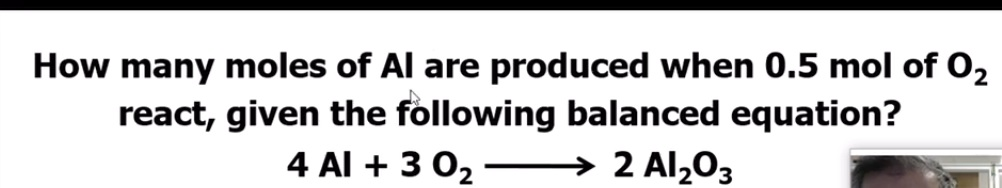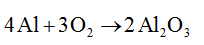# How many moles of Al are produced when 0.5 mol of O2react, given the following balanced equation?4 Al + 3 02 –→ 2 Al2O3

Question
1 views

Could you explain in detail as to how we get the correct answer? And could you explain how I am supposed to know my answer is correct afterward? (Can I use my scientific calculator or is there a formula I should know to check my answer?)

Thankshelp_outlineImage TranscriptioncloseHow many moles of Al are produced when 0.5 mol of O2 react, given the following balanced equation? 4 Al + 3 02 – → 2 Al2O3 fullscreen
check_circle

Step 1

The reaction in which the number of the atoms involved in reactants is equal to number of atoms in product is a balanced chemical reaction.

The reaction has to be balanced before the calculation.

The balanced reaction is given below....

### Want to see the full answer?

See Solution

#### Want to see this answer and more?

Solutions are written by subject experts who are available 24/7. Questions are typically answered within 1 hour.*

See Solution
*Response times may vary by subject and question.
Tagged in